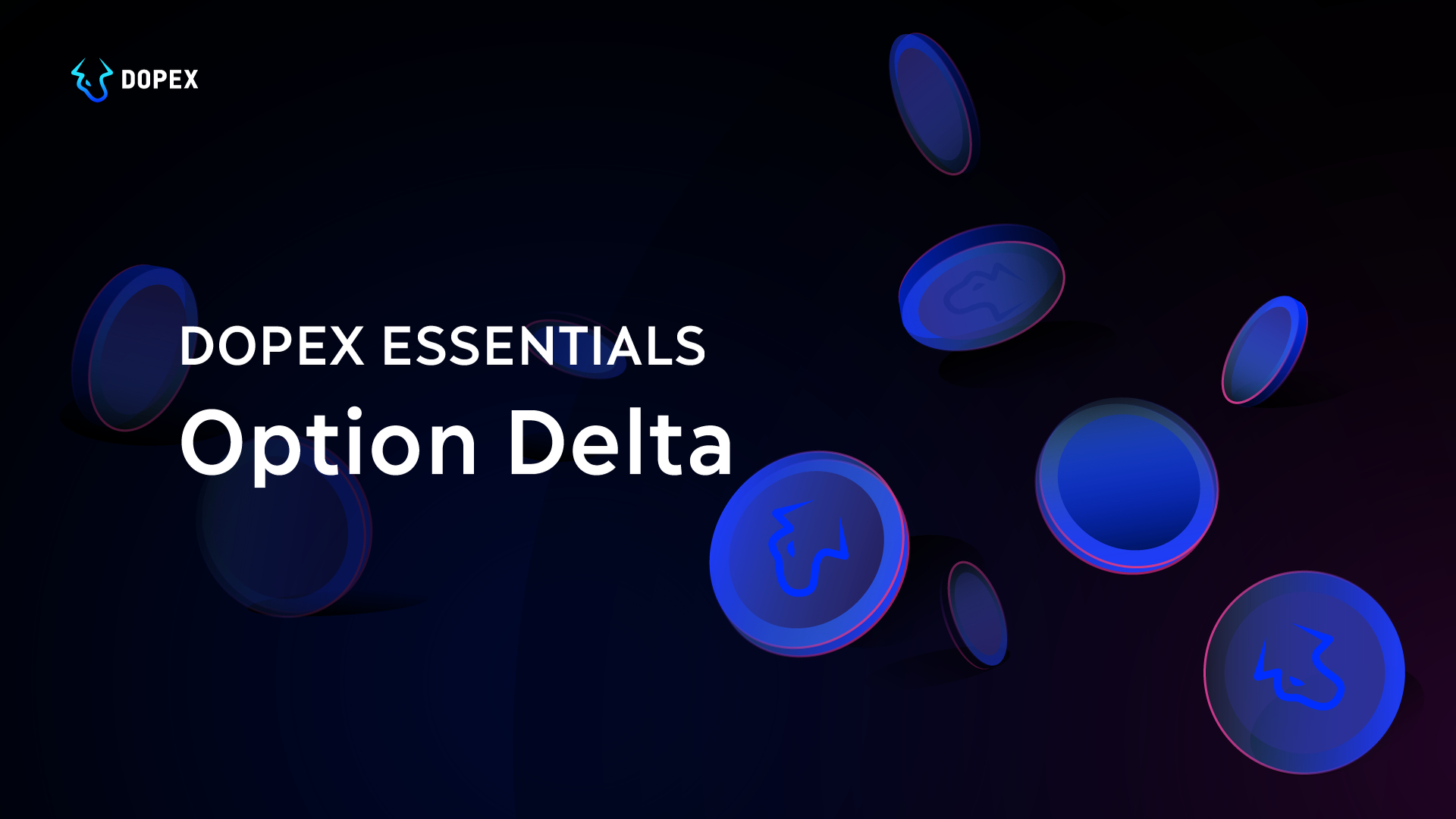# Dopex Essentials: Option Delta

Dopex-Essentials# Delta vs Underlying Asset Price

###### The chart below shows the movement of delta versus the price of the underlying asset.
1. The blue line shows the movement of a call option’s delta
2. The red line shows the movement of a put option’s delta

###### Allow me to unpack the information the chart gives us:
• As you can see by looking at the X-axis from the left to the right, the moneyness of the option increases as the price of the underlying asset moves from OTM to ATM to ITM.
• Now observe the blue line that represents the delta of a call option. We can see that when the price of the underlying asset increases, so does the delta.
• As you can see, at OTM the delta is near 0. This also means that regardless of how much the price of the underlying asset drops ( going from OTM to deep OTM) the option’s delta will remain at 0
• When the price of the underlying asset moves from OTM to ATM, the delta value also starts to increase.
• Notice how the delta lies within the 0 to 0.5 range for options that are less than ATM. And then at ATM, the delta hits a value of 0.5
• When the price of the underlying asset moves from ATM towards ITM the delta starts to move beyond the 0.5 mark.
• However, notice the delta starts to flatten out when it hits a value of 1. This also implies that when the delta moves beyond ITM to deeper ITM the delta value does not change. It remains at its maximum value of 1

# Final Conclusion

## Key Points:

1. The delta value changes along with changes in the price of the underlying asset.
2. As the option transitions from OTM to ATM to ITM, so does the delta.
3. The delta value for ATM options is 0.5
4. Delta predevelopment is when the option transitions from deep OTM to OTM.
5. Delta Takeoff and acceleration is when the option transitions from OTM to ATM.
6. Delta stabilization is when the option transitions from ATM to ITM to Deep ITM.
7. Buying options in the take-off stage tend to give high percentage returns.
8. Buying deep ITM options is as good as buying the underlying asset.

## Glossary of terms

###### In the money (ITM):
• For a call — this term is used when the strike price is lower than the current price of the underlying asset.
• For a put — this term is used when the strike is higher than the current price.
###### At the money (ATM):
• For both a call and a put — this term is used when the strike is equal to the current price.
###### Out of the money (OTM):
• For a call — this term is used when the strike price is higher than the current price of the underlying asset.
• For a put — this term is used when the strike is lower than the current price.

← Back to Blog